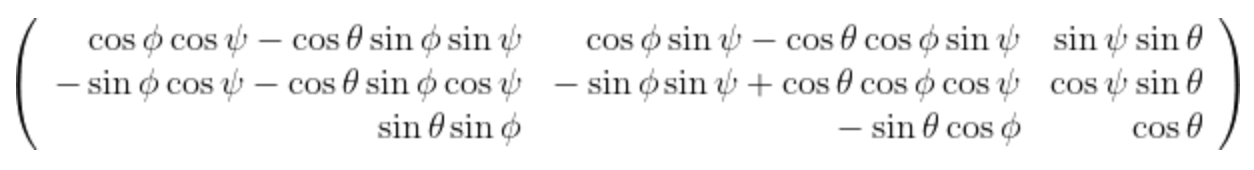### Euler angles of rotated galaxyPablo Galan de Anta
• 1 Dec '21

Hi,

I want to estimate the rotation angles I need to apply to align the galactic plane of a given galaxy in TNG50 with the cartesian axes X,Y,Z. I'm using the tensor of inertia to align the galaxy, so the transformation I've made was

``````        I[0,0] = np.sum( masses * (xyz[:,1]*xyz[:,1] + xyz[:,2]*xyz[:,2]) )
I[1,1] = np.sum( masses * (xyz[:,0]*xyz[:,0] + xyz[:,2]*xyz[:,2]) )
I[2,2] = np.sum( masses * (xyz[:,0]*xyz[:,0] + xyz[:,1]*xyz[:,1]) )
I[0,1] = -1 * np.sum( masses * (xyz[:,0]*xyz[:,1]) )
I[0,2] = -1 * np.sum( masses * (xyz[:,0]*xyz[:,2]) )
I[1,2] = -1 * np.sum( masses * (xyz[:,1]*xyz[:,2]) )
I[1,0] = I[0,1]
I[2,0] = I[0,2]
I[2,1] = I[1,2]

# get eigen values and normalized right eigenvectors
eigen_values, rotation_matrix = np.linalg.eig(I)

# sort ascending the eigen values
sort_inds = np.argsort(eigen_values)
eigen_values = eigen_values[sort_inds]

# permute the eigenvectors into this order, which is the rotation matrix which orients the
# principal axes to the cartesian x,y,z axes, such that if axes=[0,1] we have face-on
new_matrix = np.matrix( (rotation_matrix[:,sort_inds],
rotation_matrix[:,sort_inds],
rotation_matrix[:,sort_inds]) )

new_matrix = new_matrix*np.matrix( ((0,1,0),(0,0,1),(1,0,0)) )
``````

with new_matrix, the rotation matrix. Once I have the galaxy aligned, I want to recover the Euler angles theta,Psi,phi from the previous rotation to convert from the initially randomly oriented galaxy to the edge-on/face-on one. I assume the rotation matrix with the triad of angles is given byand of course, as theta can adopt 2 different values, I will have two values of phi and psi as well, however, when I'm trying to recover the initial rotation matrix with the angles I've obtained, the values I get are not even similar. It is the Euler matrix of the image not correct? Is there any easiest way to get the Euler angles?

PabloDylan Nelson
• 1 Dec '21

Assuming it is a normal rotation matrix, these instructions should work?Pablo Galan de Anta
• 2 Dec '21

I've solved the problem. One of the angles was rotated by a factor pi/2.

Thanks

• Page 1 of 1